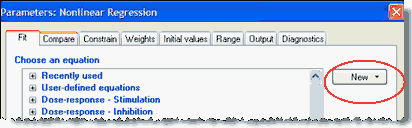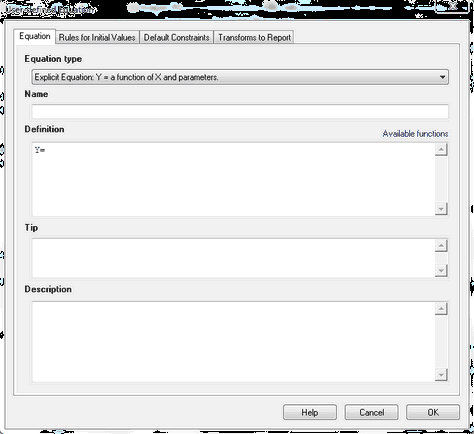## Please enable JavaScript to view this site.

 How to: Enter a new equation

## Entering an equation

Prism comes with many built-in equations, but you will often want to enter a different equation. To do so, click the New button on the top of the Fit tab of the Nonlinear regression dialog.A drop down menu lets you choose to enter a new equation, clone an existing one, or import an equation from a saved .PZF file.## Name, definition, tip and description

### Equation type

Choose whether you are entering an ordinary explicit equation (that defines Y as a function of X and parameters), an implicit equation (Y appears on both sides of the equals sign) or a differential equation (which defines the derivative of Y with respect to X).

### Name

Enter a name you will recognize, so you can choose the equation again. The name also appears on the results table, so make it both descriptive and concise.

### Definition

Write the math that defines Y as a function of X and one or more parameters. Read the details of equation syntax, a list of available math functions you can use, and how to fit different models to different ranges of X values and to different data sets.

### Tip

The tip appears below the equation list in the Fit tab of the Nonlinear regression dialog. Use it to distinguish among similar equations. Tips are optional, and must be short (a few sentences).

### Description

The description can be up to several paragraphs. Anyone choosing the equation can view the description (and the math) by clicking the Edit or View button. This is a good place to document the source of the equation, its assumptions, units of the parameters, etc.

## Rules for initial values

Nonlinear regression must begin with initial estimated values for each parameter. You can enter values directly, or enter a rule whereby Prism can compute the initial value from the range of the X and Y values. Learn more.

The values or rules you enter will become the default set of initial values that will be used every time the equation is selected. But each time the equation is selected, you (or whoever is selecting the equation) can change the initial values for that one fit.

## Default constraints

Use the constraints tab to set default constraints. You can constrain a parameter to a constant value, constrain to a range of values, share among data sets (global fit), or define a parameter to be a column constant. These constraints will become the default every time the equation is selected. But each time the equation is selected, you (or whoever is selecting the equation) can change the constraints for that one fit.

If a parameter has to be set constant, but the actual value is different for each experiment, set the constraint "Constant equal to" but leave the value blank. If someone chooses the equation but forgets to constrain that parameter to a constant value, Prism will prompt for one.

## Transforms to report

Define transforms of the best-fit values on the Transforms to report tab. Unlike initial values and constraints, you can not override these transforms each time you choose the equation. Transforms are defined with the equation definition and can not be tweaked each time the equation is selected.